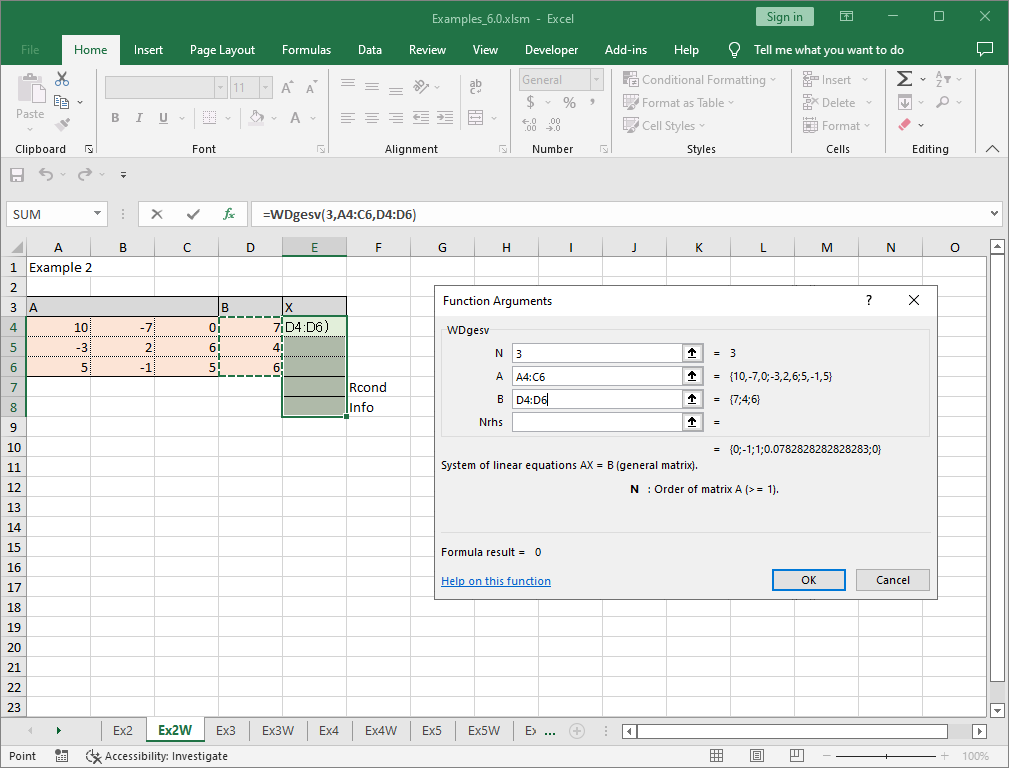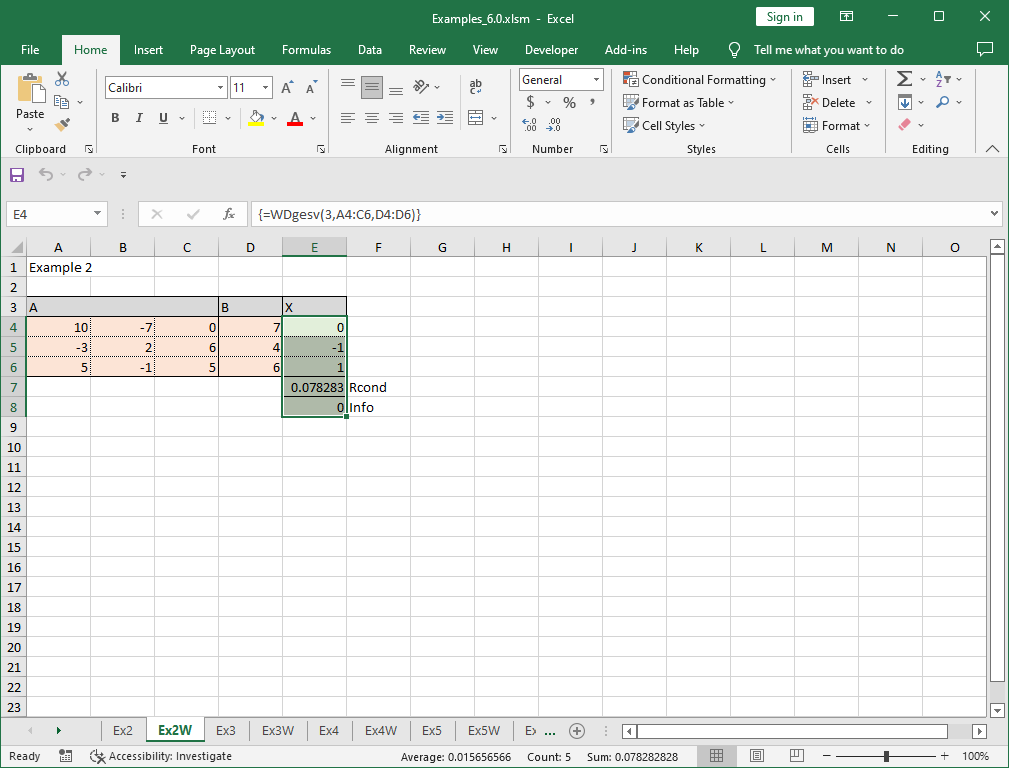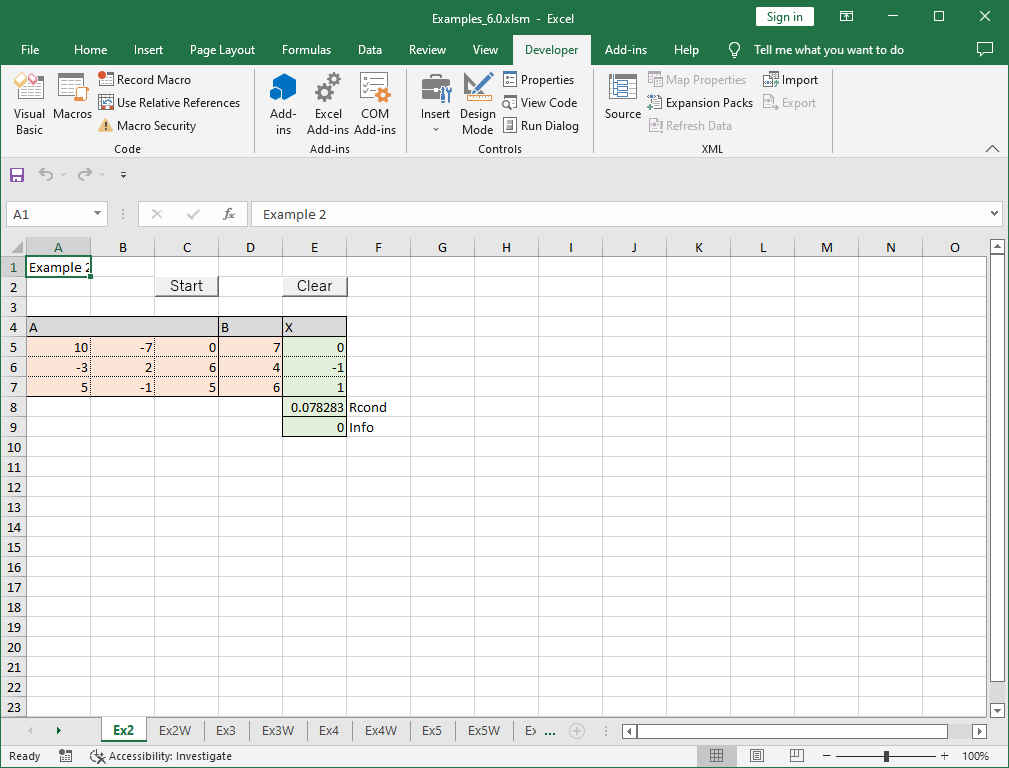# 2. System of linear equations

Note – The example worksheets are available for download. See 1. How to obtain and use example worksheets.

Examples of solving systems of linear equations by XLPack are shown below.

### Example

Now, we will solve the following linear equations.

```
10x1 - 7x2 = 7
-3x1 + 2x2 + 6x3 = 4
5x1 - x2 + 5x3 = 6

```

These equations are conveniently written in the matrix form.

```
Ax = b

```

where,

```
( 10 -7  0 )        ( 7 )        ( x1 )
A = ( -3  2  6 ),   b = ( 4 ),   x = ( x2 )
(  5 -1  5 )        ( 6 )        ( x3 )

```

A is the coefficient matrix, b is the right-hand side vector, and x is the solution vector.

Two solving methods using worksheet function WDgesv and VBA subroutine Dgesv are shown below.

### Solving problem with worksheet function

Enter the data of coefficient matrix A and right-hand side vector b into the appropriate cells (orange cells) in the worksheet, and select (order of the equations + 2) cells where the solution vector is to be placed (green cells) and enter woeksheet function WDgesv.

The parameters required by WDgesv are N, A, B and Nrhs. N is the order of the equations (e.g. 3), A is the cell range of the coefficient matrix A, and B is the cell range of the right-hand side vector b. The right-hand side vector is one in most cases. However, more than one vectors can be entered and Nrhs is the number of right-hand side vectors. Nrhs can be omitted, in which case it is assumed to be one.Then press Ctrl + Shift + Enter.Now, we’ve got the solution X=(0 -1 1)T. The next cell with the value of 0.078283 is the reciprocal of the the estimated condition number and the undermost cell with the value 0 is the return code (0 shows normal return).

### Solving problem with VBA program

Let’s solve the same example problem by VBA program. The following is an example code using VBA library subroutine Dgesv.

``````Sub Start()
Const NMax = 10
Dim N As Long, A(NMax, NMax) As Double, B(NMax) As Double
Dim Anorm As Double, RCond As Double
Dim Ipiv(NMax) As Long, Info As Long, I As Long, J As Long
'--- Input data
N = 3
For I = 0 To N - 1
For J = 0 To N - 1
A(I, J) = Cells(5 + I, 1 + J)
Next
B(I) = Cells(5 + I, 4)
Next
'--- Solve equation and compute condition number
Anorm = Dlange("1", N, N, A())
Call Dgesv(N, A(), Ipiv(), B(), Info)
If Info = 0 Then
Call Dgecon("1", N, A(), Anorm, RCond, Info)
End If
'--- Output solution
For I = 0 To N - 1
Cells(5 + I, 5) = B(I)
Next
Cells(8, 5) = RCond
Cells(9, 5) = Info
End Sub``````

In this program, Dlange and Dgecon are used to compute a condition number. These are not necessary for just solving the equations.

After entering the data of matrix A and vector b into the appropriate cells (orange cells), the following result was obtained by running the macro “Start”. Unlike the example using worksheet functions, you cannot obtain results by simply entering the values of matrix A and vector b, and you need to run a VBA program.### Symmetric coefficient matrices

If the coefficient matrix A is symmetric, WDposv and Dposv can be used. The procedure is same as above, but only the upper or lower triangular part of the matrix A (including diagonal elements) is referred. It is not necessary to set the data in the remaining triangular part.